# Cardioid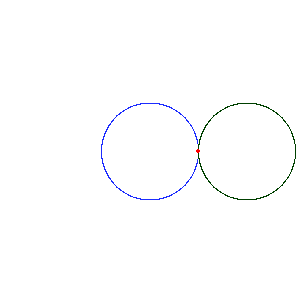A cardioid generated by a rolling circle.A cardioid given as the envelope of circles whose centers lie on a given circle and which pass through a fixed point on the given circle.

A cardioid (from the Greek καρδία "heart") is a plane curve traced by a point on the perimeter of a circle that is rolling around a fixed circle of the same radius. It can also be defined as an epicycloid having a single cusp. It is also a type of sinusoidal spiral, and an inverse curve of the parabola with the focus as the center of inversion.

The name was coined by de Castillon in 1741 but had been the subject of study decades beforehand. Named for its heart-like form, it is shaped more like the outline of the cross section of a round apple without the stalk.

A cardioid microphone exhibits an acoustic pickup pattern that, when graphed in two dimensions, resembles a cardioid, (any 2d plane containing the 3d straight line of the microphone body.) In three dimensions, the cardioid is shaped like an apple centred around the microphone which is the "stalk" of the apple.

## Equations

Based on the rolling circle description, with the fixed circle having the origin as its center, and both circles having radius a, the cardioid is given by the following parametric equations:In the complex plane this becomesHere a is the radius of the circles which generate the curve, and the fixed circle is centered at the origin. The point generating the curve touches the fixed circle at (a, 0), the cusp. The parameter t can be eliminated givingor, in rectangular coordinates,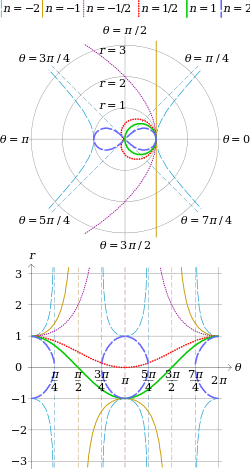Sinusoidal spirals: equilateral hyperbola (n = −2), line (n = −1), parabola (n = −1/2), cardioid (n = 1/2), circle (n = 1) and lemniscate of Bernoulli (n = 2), where rn = −1n cos in polar coordinates and their equivalents in rectangular coordinates.

These equations can be simplified somewhat by shifting the fixed circle to the right a units and choosing the point on the rolling circle so that it touches the fixed circle at the origin; this changes the orientation of the curve so that the cusp is on the left. The parametric equations are then:or, in the complex plane,With the substitution u=tan t/2,giving a rational parameterization:orThe parametrization can also be writtenand in this form it is apparent that the equation for this cardioid may be written in polar coordinates aswhere θ replaces the parameter t. This can also be writtenwhich implies that the curve is a member of the family of sinusoidal spirals.

In Cartesian coordinates, the equation for this cardioid is## Metrical properties

The area enclosed by a cardioid can be computed from the polar equation:or 6 times the area of the circles used in the construction.

The arc length of a cardioid can be computed exactly, a rarity for algebraic curves. The total length is.

## Inverse curve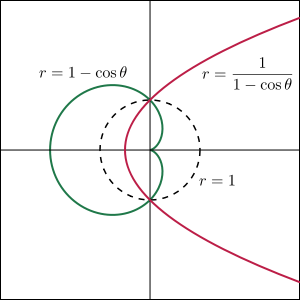The green cardioid is obtained by inverting the red parabola across the dashed circle.

The cardioid is one possible inverse curve for a parabola. Specifically, if a parabola is inverted across any circle whose center lies at the focus of the parabola, the result is a cardioid. The cusp of the resulting cardioid will lie at the center of the circle, and corresponds to the vanishing point of the parabola.

In terms of stereographic projection, this says that a parabola in the Euclidean plane is the projection of a cardioid drawn on the sphere whose cusp is at the north pole.

Not every inverse curve of a parabola is a cardioid. For example, if a parabola is inverted across a circle whose center lies at the vertex of the parabola, then the result is a cissoid of Diocles.

The picture to the right shows the parabola with polar equationIn Cartesian coordinates, this is the parabola. When this parabola is inverted across the unit circle, the result is a cardioid with the reciprocal equation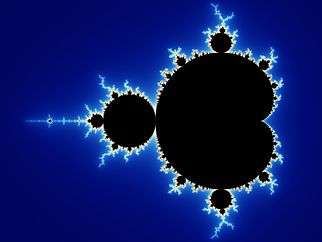Boundary of the central bulb of the Mandelbrot set is a cardioid.

## Cardioids in complex analysis

In complex analysis, the image of any circle through the origin under the mapis a cardioid. One application of this result is that the boundary of the central bulb of the Mandelbrot set is a cardioid given by the equationThe Mandelbrot set contains an infinite number of slightly distorted copies of itself and the central bulb of any of these smaller copies is an approximate cardioid.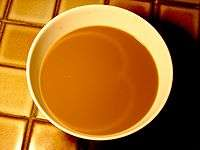The caustic appearing on the surface of this cup of coffee is a cardioid.

## Caustics

Certain caustics can take the shape of cardioids. The catacaustic of a circle with respect to a point on the circumference is a cardioid. Also, the catacaustic of a cone with respect to rays parallel to a generating line is a surface whose cross section is a cardioid. This can be seen, as in the photograph to the right, in a conical cup partially filled with liquid when a light is shining from a distance and at an angle equal to the angle of the cone. The shape of the curve at the bottom of a cylindrical cup is half of a nephroid, which looks quite similar.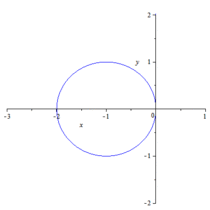Generating a cardioid using a circle and tangent linesWikimedia Commons has media related to Cardioids.